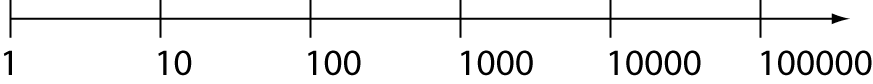Definition of Logarithmic ScaleA logarithmic scale is a non-linear scale that is used when your data has a large range of values.

The range of values between consecutive marks on the scale increases by a factor of $b$, where $b$ is the base of the logarithm.

$10$ is the most commonly used base for logarithmic scales. The scale shown above is a base $10$ logarithmic scale.

Logarithmic scales are used to record earthquake strength (the Richter scale is logarithmic), and sound intensity. Other uses include recording the intensity of light and the pH of solutions.

Description

The aim of this dictionary is to provide definitions to common mathematical terms. Students learn a new math skill every week at school, sometimes just before they start a new skill, if they want to look at what a specific term means, this is where this dictionary will become handy and a go-to guide for a student

Audience

Year 1 to Year 12 students

Learning Objectives

Learn common math terms starting with letter L

Author: Subject Coach